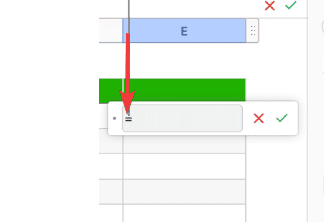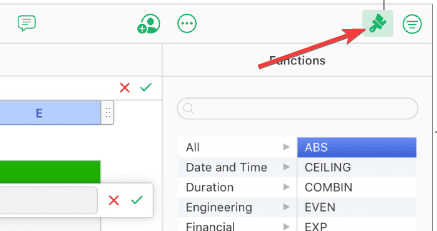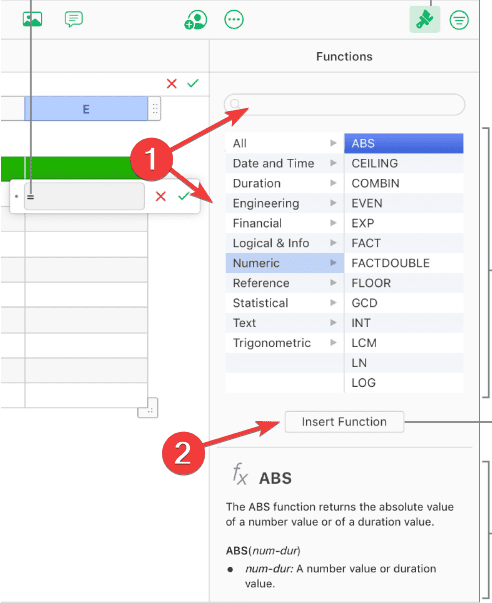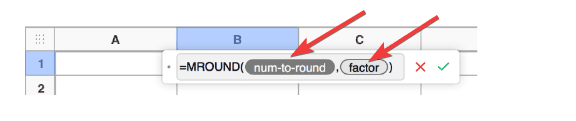# How to Write Formulas in Apple Numbers and MoreNumbers is one of the most powerful spreadsheet tools available due to its intuitive design, excellent functionality, and iCloud syncing. It includes over 290 predefined functions that help you create formulas, and provides the option to design your own using mathematical operators. Follow along with us to find out how to write formulas in Apple Numbers.

## Are Apple Numbers Formulas the Same as Excel?

The goal of Apple’s development of the spreadsheet program Numbers, which was included as part of the iWork productivity suite along with Keynote and Pages, was to simplify the process of creating spreadsheets that are neatly organized and present themselves in a professional manner.

Numbers operates in a manner that is considerably distinct from that of typical spreadsheets such as Microsoft Excel. Therefore, despite the fact that they may share the bulk of the functions, which are operations that you can include in a formula, the two applications do have different styles. Numbers also features a large number of aesthetic changes designed to enhance the visual appeal of spreadsheets.

## Can You Do Formulas in Numbers?

Yes, you can! Data manipulation, calculations, information retrieval and more may all be carried out with the help of any one of more than 290 preset functions. Also, by using the addition (+), subtraction (-), multiplication (*), and division (/) mathematical operators, you are able to create your own formula.

There are other predefined functions for engineering, economics, and statistics; some of these functions obtain information remotely through the Internet even.

## How Do You Write Formulas in Apple Numbers?

Time needed: 3 minutes.

1. Select the cell where you want the result to be displayed, then type the equal sign (=). The Formula editor will open. You can move it by dragging it from the left side.2. Select any cell that you want to include in your formula, or type a value.

3. Next, type an arithmetic operator. These include +, , *, and /. You can also choose another cell to use in your formula, or again, type a value.

4. You can add as many mathematical operators and cell references as you want, then press Return in order to see the result.

5. By clicking the Cancel button, you will exit the formula editor without saving any changes. The Syntax Error icon will display in the result cell if your formula contains a mistake. To view the error message, click the icon.

6. In order to use predefined functions, type an equal sign (=). The Functions Browser will appear in the sidebar.

7. Make sure that the Format button is selected.8. Type the name of the function that you want to use, or explore the list of functions. Double-click the one that you want to add or click the Insert Function button above the information box. It will include all you need to know about the function.9. The function will now appear along with its mandatory and optional parameters. Select a parameter then enter a value or choose which cell(s) to be included.This is how to do calculations in Apple Numbers.

### Does Apple Numbers have a Formula Bar?

No, Numbers does not have a Formula Bar as you would anticipate if you have ever used Microsoft Excel; rather, it has a floating Formula Bar that appears whenever you add the equal sign (=) in a cell.

Alternatively, you can also select a cell, click the Insert button and choose New Formula.

### How Do I Apply a Formula to an Entire Column in Numbers?

Using the AutoFill handle is one of the most straightforward approaches to applying a formula to an entire column. Thus do the following:

1. Select the cell that contains the formula you want to copy, then slide your cursor over the cell’s border until a yellow autofill handle (a dot) appears.
2. Drag the handle over those cells that you want to have the same formula as the original one.(Image Credit: Apple)

## Can You Do Equations in Apple Numbers?

You have the option of adding mathematical expressions and equations to a spreadsheet in the form of floating objects. In order to include an equation in your spreadsheet, first you will need to construct it in the Numbers equation dialog by making use of LaTeX instructions or MathML components, and then you will need to enter it.

You also have the option of inserting equations directly into text boxes and shapes, where they will appear in line with the content.

1. Select the cell or place where you want to insert your equation.
2. Select Insert > Equation.
3. Use LaTeX commands or MathML elements to insert the equation you desire.
4. Click Insert.

The three most typical general uses for spreadsheet software are the creation of budgets, the production of graphs and charts, and the storage and sorting of data, but you are free to use the spreadsheet program however you see fit, especially now that you have a working knowledge of formulas and functions.

This brings an end to our article on how to write formulas in Apple Numbers. For more interesting information, you can check out our guide on how to automatically sequence dates with Numbers. Be sure to share your ideas with us by leaving a comment in the dedicated section below!

This site uses Akismet to reduce spam. Learn how your comment data is processed.